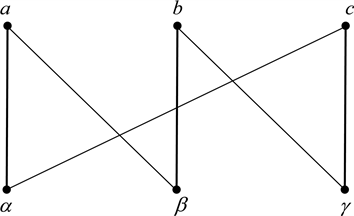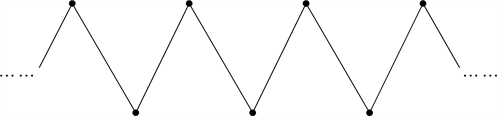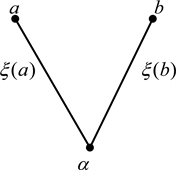﻿ 非对易量子引力

# 非对易量子引力Noncommutative Quantum Gravity

Abstract: To solve the difficulty of quantization in gravitational field, we introduce a very different approach to the theory of gravitational field. This paper is based on the principle of equivalence and the un-certainty principle. We find that introducing the uncertainty principle into the inertial coordinate system will change the geometry of spacetime, and the geometry of the gravitational field has be-come the noncommutative lattices. Then the gravitational field appears in the sense of the general relativity. We obtain the semiclassical graviton. We discuss the dynamics and quantization of the graviton, and obtain the gravitational field equation. We obtain the Green’s function of the graviton by the field equation, and the resulting Feynman rule can solve the difficulty of the Feynman integral divergence.

1. 引言

2. 非对易格

${S}^{1}=\left\{0\le \varphi \le 2\text{π},\text{\hspace{0.17em}}\mathrm{mod}\text{\hspace{0.17em}}2\text{π}\right\}$${S}^{1}$ 上粒子探测器 ${U}_{1},{U}_{2},{U}_{3}$ 各自的测量范围如下：

${U}_{1}=\left(-\frac{1}{3}\text{π},\frac{2}{3}\text{π}\right),\text{\hspace{0.17em}}{U}_{2}=\left(\frac{1}{3}\text{π},\frac{4}{3}\text{π}\right),\text{\hspace{0.17em}}{U}_{3}=\left(\text{π},2\text{π}\right)$ (2.1)

$\begin{array}{l}{U}_{1}\cap {U}_{3}=\left\{\frac{5}{3}\text{π}<\varphi <2\text{π}\right\}\to \alpha \\ {U}_{1}\cap {U}_{2}=\left\{\frac{1}{3}\text{π}<\varphi <\frac{2}{3}\text{π}\right\}\to \beta \\ {U}_{2}\cap {U}_{3}=\left\{\text{π}<\varphi <\frac{4}{3}\text{π}\right\}\to \gamma \end{array}$ (2.2)

$\begin{array}{l}{U}_{1}\\left\{{U}_{1}\cap {U}_{2}\cup {U}_{1}\cap {U}_{2}\right\}=\left\{0\le \varphi \le \frac{1}{3}\text{π}\right\}\to a\\ {U}_{2}\\left\{{U}_{2}\cap {U}_{1}\cup {U}_{2}\cap {U}_{3}\right\}=\left\{\frac{2}{3}\text{π}\le \varphi \le \text{π}\right\}\to b\\ {U}_{3}\\left\{{U}_{3}\cap {U}_{2}\cup {U}_{3}\cap {U}_{1}\right\}=\left\{\frac{4}{3}\text{π}\le \varphi \le \frac{5}{3}\text{π}\right\}\to c\end{array}$

3. 非对易格与引力场Figure 1. Hasse diagram of lattices ${P}_{6}\left(S1\right)$Figure 2. Hasse diagram of lattices $P\left(R1\right)$Figure 3. $\vee$ poset

Planck时间 ${t}_{p}$ 的精度，因此在引力场中我们试取此测不准区域的半径为Planck长度 ${l}_{p}$ 和Planck时间 ${t}_{p}$$\left(\Delta r,\Delta t\right)=\left({l}_{p},{t}_{p}\right)$

$\lambda \left(\stackrel{^}{\xi }\left(\vee \right)\right)=\xi \left(a\right)\text{\hspace{0.17em}}\text{or}\text{\hspace{0.17em}}\xi \left(b\right)$ (3.1)

${l}_{p}\to {l}_{p}\cdot \frac{\text{d}\xi \left(x\right)}{\text{d}x}$ (3.2)

$\xi \left(b\right)=\xi \left(a\right)±{l}_{p}\cdot {\frac{\text{d}\xi \left(x\right)}{\text{d}x}|}_{x=a}$ (3.3)

${\lambda \left(\stackrel{^}{\xi }\right)|}_{X}=\xi \left(X\right)±{l}_{p}\cdot {\frac{\text{d}\xi \left(x\right)}{\text{d}x}|}_{x=X}$ (3.4)

$\begin{array}{l}{x}^{1}=r\mathrm{sin}\theta \mathrm{cos}\varphi \\ {x}^{2}=r\mathrm{sin}\theta \mathrm{sin}\varphi \\ {x}^{3}=r\mathrm{cos}\theta \\ {x}^{4}=t\end{array}$ (3.5)

4维球极坐标系中的Minkowski度规为：

${\eta }_{ij}=\left(\begin{array}{cccc}1& & & \\ & {r}^{2}& & \\ & & {r}^{2}{\mathrm{sin}}^{2}\theta & \\ & & & -1\end{array}\right)$ (3.6)

4维Minkowski空时中的整体惯性系算符记为 $\stackrel{^}{\xi }$ 。引入测不准原理后，在球极坐标系 ${r}^{i}$ 的极点x处的惯性系可在 ${r}^{i}$ 中表示为：

$\lambda \left(\stackrel{^}{\xi }\right)=\left(\lambda \left({\stackrel{^}{\xi }}^{r}\right),\lambda \left({\stackrel{^}{\xi }}^{\theta }\right),\lambda \left({\stackrel{^}{\xi }}^{\varphi }\right),\lambda \left({\stackrel{^}{\xi }}^{t}\right)\right)$ (3.7)

$\begin{array}{l}\lambda \left({\stackrel{^}{\xi }}^{r}\right)={\xi }^{r}+{l}_{p}\cdot \frac{\partial {\xi }^{r}}{\partial r}\\ \lambda \left({\stackrel{^}{\xi }}^{\theta }\right)={\xi }^{\theta }\\ \lambda \left({\stackrel{^}{\xi }}^{\varphi }\right)={\xi }^{\varphi }\\ \lambda \left({\stackrel{^}{\xi }}^{t}\right)={\xi }^{t}+{t}_{p}\cdot \frac{\partial {\xi }^{t}}{\partial t}\end{array}$ (3.8)

$\begin{array}{l}\lambda \left({\stackrel{^}{\xi }}^{r}\right)={\xi }^{r}+{l}_{p}\cdot \frac{\partial {\xi }^{r}}{\partial r}=r\\ \lambda \left({\stackrel{^}{\xi }}^{\theta }\right)={\xi }^{\theta }=\theta \\ \lambda \left({\stackrel{^}{\xi }}^{\varphi }\right)={\xi }^{\varphi }=\varphi \\ \lambda \left({\stackrel{^}{\xi }}^{t}\right)={\xi }^{t}+{t}_{p}\cdot \frac{\partial {\xi }^{t}}{\partial t}=t\end{array}$ (3.9)

${\xi }^{i}=\left\{\begin{array}{l}{\xi }^{r}=\left(r-{l}_{p}\right)+{C}_{r}\mathrm{exp}\left(-\frac{r}{{l}_{p}}\right)+{{C}^{\prime }}_{r}\\ {\xi }^{\theta }=\theta +{{C}^{\prime }}_{\theta }\\ {\xi }^{\varphi }=\varphi +{{C}^{\prime }}_{\varphi }\\ {\xi }^{t}=\left(r-{t}_{p}\right)+{C}_{t}\mathrm{exp}\left(-\frac{|t|}{{t}_{p}}\right)+{{C}^{\prime }}_{t}\end{array}$ (3.10)

${\xi }^{i}=\left\{\begin{array}{l}{\xi }^{r}=r+{C}_{r}\mathrm{exp}\left(-\frac{r}{{l}_{p}}\right)\\ {\xi }^{\theta }=\theta \\ {\xi }^{\varphi }=\varphi \\ {\xi }^{t}=t+{C}_{t}\mathrm{exp}\left(-\frac{|t|}{{t}_{p}}\right)\end{array}$ (3.11)

$C=\frac{1}{2\Delta x}$ , 则当 $\Delta x\to 0$ 时函数 $C\mathrm{exp}\left(-\frac{x}{\Delta x}\right)$ 为Dirac-δ函数。由于半径 $\left({l}_{p},{t}_{p}\right)$ 是非常小量，因此式(3.11)近似于4维Dirac-δ函数，所以方程组(3.9)的解可以视为球极坐标系极点处的一个粒子。由于式(3.11)表示极点处的局部惯性系，因此此粒子可理解为位于极点处的一个半经典的引力子。

$\begin{array}{c}{g}_{ij}\left(r\right)={\eta }_{mn}\frac{\partial {\xi }^{m}}{\partial {r}^{i}}\frac{\partial {\xi }^{n}}{\partial {r}^{j}}\\ =\left(\begin{array}{cccc}{\left(1-\frac{{C}_{r}}{{l}_{p}}\mathrm{exp}\left(-\frac{r}{{l}_{p}}\right)\right)}^{2}& & & \\ & {r}^{2}& & \\ & & {r}^{2}{\mathrm{sin}}^{2}\theta & \\ & & & -{\left(1-\frac{{C}_{t}}{{t}_{p}}\mathrm{exp}\left(-\frac{|t|}{{t}_{p}}\right)\right)}^{2}\end{array}\right)\end{array}$ (3.12)

4. 引力子的动力学与量子化

${\xi }^{i}\left(\tau ,r\right)=\left\{\begin{array}{l}{\xi }^{r}=r+{C}_{r}\left(\tau \right)\mathrm{exp}\left(-\frac{r}{{l}_{p}}\right)\\ {\xi }^{\theta }=\theta \left(\tau \right)\\ {\xi }^{\varphi }=\varphi \left(\tau \right)\\ {\xi }^{t}=t+{C}_{t}\left(\tau \right)\mathrm{exp}\left(-\frac{|t|}{{t}_{p}}\right)\end{array}$ (4.1)

$\begin{array}{c}S=m\int \text{d}s\\ =m\int \text{d}\tau \sqrt{-{\eta }_{ij}\frac{\partial {\xi }^{i}}{\partial \tau }\frac{\partial {\xi }^{j}}{\partial \tau }}\\ \equiv m\int \text{d}\tau \sqrt{-{\eta }_{ij}{\stackrel{˙}{\xi }}^{i}{\stackrel{˙}{\xi }}^{j}}\end{array}$ (4.2)

Lagrange密度为：

$L=m\cdot \sqrt{-{\eta }_{ij}{\stackrel{˙}{\xi }}^{i}{\stackrel{˙}{\xi }}^{j}}\equiv m\cdot \sqrt{-{\stackrel{˙}{\xi }}^{i}{\stackrel{˙}{\xi }}_{i}}$ (4.3)

Lagrange方程为:

${\partial }_{\tau }\left(\frac{\partial L}{\partial {\stackrel{˙}{\xi }}^{i}}\right)=\frac{\partial L}{\partial {\xi }^{i}}$ (4.4)

$\frac{\partial L}{\partial {\xi }^{i}}=0$ (4.5)

${\partial }_{\tau }\left(\frac{\partial L}{\partial {\stackrel{˙}{\xi }}^{i}}\right)={\partial }_{\tau }\left[m\cdot \frac{{\stackrel{˙}{\xi }}^{i}}{\sqrt{-{\stackrel{˙}{\xi }}^{2}}}\right]=0$ (4.6)

$\begin{array}{c}S=-\frac{1}{2}\int \text{d}\tau \sqrt{{g}_{\tau \tau }}\left({g}^{\tau \tau }{\stackrel{˙}{\xi }}^{\text{i}}{\stackrel{˙}{\xi }}^{j}{\eta }_{ij}-{m}^{2}\right)\\ =-\frac{1}{2}\int \text{d}\tau \text{ }e\cdot \left(\frac{{\stackrel{˙}{\xi }}^{2}}{{e}^{2}}-{m}^{2}\right)\end{array}$ (4.7)

$\delta S=\frac{1}{2}\int \text{d}\tau \left(\frac{{\stackrel{˙}{\xi }}^{2}}{{e}^{2}}+{m}^{2}\right)\delta e$ (4.8)

$\delta S=0$ 可以给出对 $e\left(\tau \right)$ 的运动方程：

$\frac{{\stackrel{˙}{\xi }}^{2}}{{e}^{2}}+{m}^{2}=0$ (4.9)

$e=\frac{\sqrt{-{\stackrel{˙}{\xi }}^{2}}}{m}$ (4.10)

$\delta S=\frac{1}{2}\int \text{d}\tau \left(\frac{2{\stackrel{˙}{\xi }}^{i}}{{e}^{2}}\right){\partial }_{\tau }\delta {\xi }^{i}$ (4.11)

${\partial }_{\tau }\left({e}^{-1}{\stackrel{˙}{\xi }}^{i}\right)=0$ (4.12)

${\partial }_{\tau }{\stackrel{˙}{\xi }}^{i}-{e}^{-1}\stackrel{˙}{e}{\stackrel{˙}{\xi }}^{i}=0$ (4.13)

${\partial }^{\tau }{\partial }_{\tau }{\xi }^{i}=0$ (4.14)

${\xi }^{i}\left(x,r\right)=\left\{\begin{array}{l}{\xi }^{r}=r+{C}_{r}\left(x\right)\mathrm{exp}\left(-\frac{r}{{l}_{p}}\right)\\ {\xi }^{\theta }=\theta \left(x\right)\\ {\xi }^{\varphi }=\varphi \left(x\right)\\ {\xi }^{t}=t+{C}_{t}\left(x\right)\mathrm{exp}\left(-\frac{|t|}{{t}_{p}}\right)\end{array}$ (4.15)

${\partial }^{\mu }{\partial }_{\mu }{\xi }^{i}=0$ (4.16)

${\xi }^{i}\left(x,r\right)=\left\{\begin{array}{l}{\xi }^{r}=r+〈x|{C}_{r}〉\mathrm{exp}\left(-\frac{r}{{l}_{p}}\right)\\ {\xi }^{\theta }=〈x|\theta 〉\\ {\xi }^{\varphi }=〈x|\varphi 〉\\ {\xi }^{t}=t+〈x|{C}_{t}〉\mathrm{exp}\left(-\frac{|t|}{{t}_{p}}\right)\end{array}$ (4.17)

$\begin{array}{l}〈x|{C}_{r}〉=\int {\text{d}}^{4}k\left({C}_{r}\left(k\right)\mathrm{exp}\left(ikx\right)+{C}_{r}^{*}\left(k\right)\mathrm{exp}\left(-ikx\right)\right)\\ 〈x|\theta 〉=\int {\text{d}}^{4}k\left(\theta \left(k\right)\mathrm{exp}\left(ikx\right)+{\theta }^{*}\left(k\right)\mathrm{exp}\left(-ikx\right)\right)\\ 〈x|\varphi 〉=\int {\text{d}}^{4}k\left(\varphi \left(k\right)\mathrm{exp}\left(ikx\right)+{\varphi }^{*}\left(k\right)\mathrm{exp}\left(-ikx\right)\right)\\ 〈x|{C}_{t}〉=\int {\text{d}}^{4}k\left({C}_{t}\left(k\right)\mathrm{exp}\left(ikx\right)+{C}_{t}^{*}\left(k\right)\mathrm{exp}\left(-ikx\right)\right)\end{array}$ (4.18)

$\begin{array}{l}\left[{C}_{r}\left(k\right),{C}_{r}^{*}\left({k}^{\prime }\right)\right]=\delta \left(k-{k}^{\prime }\right)\\ \left[\theta \left(k\right),{\theta }^{*}\left({k}^{\prime }\right)\right]=\delta \left(k-{k}^{\prime }\right)\\ \left[\varphi \left(k\right),{\varphi }^{*}\left({k}^{\prime }\right)\right]=\delta \left(k-{k}^{\prime }\right)\\ \left[{C}_{t}\left(k\right),{C}_{t}^{*}\left({k}^{\prime }\right)\right]=\delta \left(k-{k}^{\prime }\right)\end{array}$ (4.19)

$L=-\frac{1}{2}\left({\eta }^{\mu \nu }\frac{\partial {\xi }^{i}}{\partial {x}^{\mu }}\frac{\partial {\xi }^{j}}{\partial {x}^{\nu }}{\eta }_{ij}-{m}^{2}\right)$ (4.20)

${\xi }^{i}$ 的共轭动量为：

${p}_{i}=\frac{\partial L}{\partial {\stackrel{˙}{\xi }}^{i}}=-{\stackrel{˙}{\xi }}^{j}{\eta }_{ij}=-{\stackrel{˙}{\xi }}_{i}$ (4.21)

Hamilton密度为：

$H=\frac{\partial L}{\partial {\stackrel{˙}{\xi }}^{i}}{\stackrel{˙}{\xi }}^{i}-L=-\frac{1}{2}\left(\nabla {\xi }^{i}\nabla {\xi }^{j}{\eta }_{ij}+{p}_{\mu }{p}^{\mu }+{m}^{2}\right)$ (4.22)

Hamilton量为：

$H=\int {\text{d}}^{3}x\text{\hspace{0.17em}}H$ (4.23)

$\begin{array}{c}{T}_{\mu \nu }={\eta }_{\mu \nu }L-\frac{\partial L}{\partial \left({\partial }^{\mu }{\xi }^{i}\right)}{\partial }_{\nu }{\xi }^{i}\\ =\left(\frac{{\eta }_{\mu \nu }}{2}\left({\partial }^{\lambda }{\xi }^{i}{\partial }_{\lambda }{\xi }^{j}{\eta }_{ij}+{m}^{2}\right)-{\partial }_{\mu }{\xi }^{i}{\partial }_{\nu }{\xi }^{i}{\eta }_{ij}\right)\end{array}$ (4.24)

5. 引力子的Green函数

${R}^{\mu }\left(X\right)=\left({l}_{p}^{1}\left(X\right),{l}_{p}^{2}\left(X\right),{l}_{p}^{3}\left(X\right),{l}_{p}^{4}\left(X\right)\right)$

$\begin{array}{l}{l}_{p}^{1}\left(X\right)={l}_{p}|\mathrm{sin}\theta \left(X\right)\mathrm{cos}\varphi \left(X\right)|\\ {l}_{p}^{2}\left(X\right)={l}_{p}|\mathrm{sin}\theta \left(X\right)\mathrm{sin}\varphi \left(X\right)|\\ {l}_{p}^{3}\left(X\right)={l}_{p}|\mathrm{cos}\theta \left(X\right)|\\ {l}_{p}^{1}\left(X\right)={t}_{p}\end{array}$ (5.1)

${C}^{\mu }\left(X\right)=\left({C}^{1}\left(X\right),\text{\hspace{0.17em}}{C}^{2}\left(X\right),\text{\hspace{0.17em}}{C}^{3}\left(X\right),\text{\hspace{0.17em}}{C}^{4}\left(X\right)\right)$

$\begin{array}{l}{C}^{1}\left(x\right)={C}_{r}\left(X\right)\mathrm{sin}\theta \left(X\right)\mathrm{cos}\varphi \left(X\right)\\ {C}^{2}\left(x\right)={C}_{r}\left(X\right)\mathrm{sin}\theta \left(X\right)\mathrm{sin}\varphi \left(X\right)\\ {C}^{3}\left(x\right)={C}_{r}\left(X\right)\mathrm{cos}\theta \left(X\right)\\ {C}^{}\left(x\right)={C}_{t}\left(X\right)\end{array}$ (5.2)

${\xi }^{\mu }\left(X,x\right)={x}^{\mu }+{C}^{\mu }\left(X\right)\mathrm{exp}\left(-\frac{|{x}^{\mu }|}{{R}^{\mu }\left(X\right)}\right)$ (5.3)

$\square \text{ }{\xi }^{\mu }\left(X,x\right)={j}^{\mu }\left(X\right)$ (5.4)

$\frac{|{x}^{\mu }|}{{R}^{\mu }\left(X\right)}$ 简记为 $\stackrel{¯}{R}\left(x\right)$ 。由于 ${R}^{\mu }\left(X\right)$ 只是常数 $\left({l}_{p},\text{\hspace{0.17em}}{t}_{p}\right)$ 在正交坐标系 ${X}^{\mu }$ 中的分量形式，所以 $\stackrel{¯}{R}\left(x\right)$ 可以简单理解为引力子波包延展结构的变量x的函数（即原来球极坐标系中变量r的函数），因此D'Alembert算子不作用于函数 $\stackrel{¯}{R}\left(x\right)$ 。由此场方程(5.4)可以进一步简化为如下形式：

${\text{e}}^{-\stackrel{¯}{R}\left(x\right)}\square {C}^{\mu }\left(X\right)={j}^{\mu }\left(X\right)$ (5.5)

${\text{e}}^{-\stackrel{¯}{R}\left(x\right)}\square {G}^{\mu }\left(X\right)={\delta }^{4}\left(X\right)$ (5.6)

${G}^{\mu }\left(X\right)$ 为：

$\begin{array}{l}{G}^{\mu }\left(X\right)=\frac{1}{{\left(2\text{π}\right)}^{4}}\int {\text{d}}^{4}k\text{\hspace{0.17em}}{\stackrel{˜}{G}}^{\mu }\left(k\right){\text{e}}^{-ikX}\\ {\stackrel{˜}{G}}^{\mu }\left(k\right)=\int {\text{d}}^{4}X\text{\hspace{0.17em}}{G}^{\mu }\left(x\right){\text{e}}^{ikX}\end{array}$ (5.7)

$\begin{array}{l}|x〉=\frac{1}{{\left(2\text{π}\right)}^{4}}\int {\text{d}}^{4}k\text{\hspace{0.17em}}{\text{e}}^{-ikX}|k〉\\ |k〉=\int {\text{d}}^{4}x\text{\hspace{0.17em}}{\text{e}}^{ikx}|x〉\end{array}$ (5.8)

$\square {G}^{\mu }\left(X\right)={\delta }^{4}\left(X\right){\text{e}}^{\stackrel{¯}{R}\left(x\right)}$ (5.9)

${f}_{1}={\delta }^{4}\left(X\right),\text{ }{f}_{2}={\text{e}}^{\stackrel{¯}{R}\left(x\right)}$ (5.10)

$\begin{array}{c}\stackrel{˜}{f}\left(k\right)=\int {\text{d}}^{4}X\text{\hspace{0.17em}}{f}_{1}{\text{e}}^{ikX}\cdot \int {\text{d}}^{4}x\text{\hspace{0.17em}}{f}_{2}{\text{e}}^{ikx}\\ =1\cdot \int {\text{d}}^{4}x\text{\hspace{0.17em}}{f}_{2}{\text{e}}^{ikx}=\delta \left(k-\frac{i}{{R}^{\mu }\left(X\right)}\right)\end{array}$ (5.11)

$\square \text{\hspace{0.17em}}\frac{1}{{\left(2\text{π}\right)}^{4}}\int {\text{d}}^{4}k\text{\hspace{0.17em}}{\stackrel{˜}{G}}^{\mu }\left(k\right){\text{e}}^{-ikX}=\frac{1}{{\left(2\text{π}\right)}^{4}}\int {\text{d}}^{4}k\text{\hspace{0.17em}}\stackrel{˜}{f}\left(k\right){\text{e}}^{-ikx}$ (5.12)

${\stackrel{˜}{G}}^{\mu }\left(k\right)=-\frac{\stackrel{˜}{f}\left(k\right)}{{k}^{2}}=-\frac{1}{{k}^{2}}\cdot \delta \left(k-\frac{i}{{R}^{\mu }\left(X\right)}\right)$ (5.13)

${G}^{\mu }\left(X\right)=-\frac{1}{{\left(2\text{π}\right)}^{4}}\int {\text{d}}^{4}k\text{\hspace{0.17em}}\frac{{\text{e}}^{-ikX}}{{k}^{2}}\cdot \delta \left(k-\frac{i}{{R}^{\mu }\left(X\right)}\right)$ (5.14)

${\stackrel{˜}{G}}^{i}\left(k\right)=\left\{\begin{array}{l}{\stackrel{˜}{G}}^{r}\left(k\right)=-\frac{1}{{\left({k}^{r}\right)}^{2}}\cdot \delta \left({k}^{r}-\frac{i}{{l}_{p}}\right)\\ {\stackrel{˜}{G}}^{\theta }\left(k\right)=-\frac{1}{{\left({k}^{\theta }\right)}^{2}}\\ {\stackrel{˜}{G}}^{\varphi }\left(k\right)=-\frac{1}{{\left({k}^{\varphi }\right)}^{2}}\\ {\stackrel{˜}{G}}^{t}\left(k\right)=-\frac{1}{{\omega }^{2}}\cdot \delta \left(\omega -\frac{i}{{t}_{p}}\right)\end{array}$ (5.15)

${G}^{i}\left({r}_{x}\right)=-\frac{1}{{\left(2\text{π}\right)}^{4}}\int {\text{d}}^{4}k\text{\hspace{0.17em}}{\stackrel{˜}{G}}^{i}\left(k\right)\cdot {\text{e}}^{-ik\cdot {r}_{x}}$

Green函数(5.15)是广义函数，必须在积分的意义下理解。变量 ${k}^{r}$ 的积分限为 $\left[0,+\infty \right)$ ，变量ω的积分限为 $\left(-\infty ,+\infty \right)$ 。对这两个变量的积分可以这样理解：积分路径偏离实轴进入复平面，分别经过奇点 ${k}^{r}=\frac{i}{{l}_{p}}$$\omega =\frac{i}{{t}_{p}}$ 。计算Feynman图时，根据Dirac-δ函数的性质，我们只需给出积分路径上的奇点，而不需要计算具体的积分。变量 ${k}^{\theta }$ 的积分限为 $\left[0,\text{π}\right]$ ，变量 ${k}^{\varphi }$ 的积分限为 $\left[0,2\text{π}\right)$ ，这两个变量的积分都是有限的。由此，Feynman图积分发散的困难得以解决。

6. 讨论与结论

4维Minkowski空时是一个流形，可建立整体惯性系 ${\xi }^{i}\left(x\right)$ 。由于对惯性系引入了测不准原理， ${T}_{2}$ 分离性公理不再成立，因此由惯性系张成的空间不是Hausdroff空间，而是非对易格空间，整体惯性系在非对易格空间里分解为局部惯性系。这样，由于等效原理，在广义相对论的意义上出现了引力场。因此，引力场源于测不准原理。以这样的方式引入引力相互作用可以自然地得到一个近似于Dirac-δ函数的波包作为半经典的引力子。为引力子建立作用量后可得引力子的场方程。由于引力子是一个近似于Dirac-δ函数的波包，因此由场方程推导出的引力子的Green函数可以自然地解决Feynman积分发散困难。

 Connes, A. (1994) Noncommutative Geometry. Academic Press.

 Landi, G. (1997) An Introduction to Noncommutative Spaces and Their Geometries, Lecture Notes in Physics. New Series M, Monographs No. 51, Springer-Verlag, Heidelberg.

 Weinberg, I. (1972) Gravitation and Cosmology: Principle and Applicatons of General Theory of Relativity. Wiley, New York.

 Balachandran, A.P., Bimonte, G., Ercolessi, E., Landi, G., Lizzi, F. and Parano, G. and Teotonio-Sobrinho, P. (1996) Noncommutative Lattices as Finite Approximations. Journal of Geometry and Physics, 18. hep-th/9510217

Top